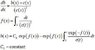# Solving differential equation

• JulieK
In summary, the conversation discusses a differential equation involving the functions b and c of x. Although there is a closed form relation between c and x, there is not one for b and x. The person is looking for a solution that links all three variables. An analytic solution can be found when c(x) is known and integrable, while a specific c(x) is needed for a nicer formula. For numerical solutions, Euler's method and Runge-Kutta are suggested as options. The formal solution is also provided in an attachment.

#### JulieK

I have the following differential equation

\begin{equation}
\frac{\partial b}{\partial x}=\frac{b-c}{c^{2}}\end{equation}

where $b$ and $c$ are both functions of $x$. However, although
I have a closed form relation between $c$ and $x$, I do not have
such a closed form relation between $b$ and $x$. Is there any analytic
or numeric way to solve this problem. I want a solution linking $b$,
$c$ and $x$

Well, when c(x) is a known (and integrable) function, then the ode for b(x) is a linear first order ODE and you can solve it by finding the integrating factor.

JulieK said:
I have the following differential equation

\begin{equation}
\frac{\partial b}{\partial x}=\frac{b-c}{c^{2}}\end{equation}

where $b$ and $c$ are both functions of $x$. However, although
I have a closed form relation between $c$ and $x$, I do not have
such a closed form relation between $b$ and $x$. Is there any analytic
or numeric way to solve this problem. I want a solution linking $b$,
$c$ and $x$

Hi JulieK!Wolfram|Alpha gives this analytic solution.
To get a nicer formula, you need a specific c(x).

To solve numerically, the simplest method you can use is Euler's method.
Euler's method uses that:
$$db=\frac{b-c}{c^{2}}dx$$
From a given ##x_0## and ##b_0##, and with a stepsize ##h##, the algorithm is:
\left[ \begin{align}x_{n+1} &= x_n + h \\ b_{n+1} &= b_n + h \frac{b_n-c(x_n)}{c(x_n)^{2}} \end{align} \right.

A more advanced and accurate method is Runge-Kutta, which is described here.

The formal solution is in attachment :

#### Attachments

•EDOlin.JPG
8.5 KB · Views: 385